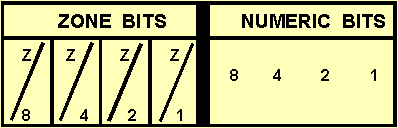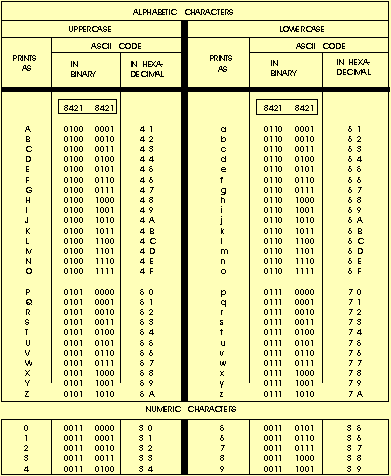Computer coding systemsCustom SearchCOMPUTER CODING SYSTEMS

To represent numeric, alphabetic, and special characters in a computer's internal storage and on magnetic media, we must use some sort of coding system. In computers, the code is made up of fixed size groups of binary positions. Each binary position in a group is assigned a specific value; for example 8, 4, 2, or 1. In this way, every character can be represented by a combination of bits that is different from any other combination.

In this section you will learn how the selected coding systems are used to represent data. The coding systems included are Extended Binary Coded Decimal Interchange Code (EBCDIC), and American Standard Code for Information Interchange (ASCII).

EXTENDED BINARY CODED DECIMAL INTERCHANGE CODE (EBCDIC)

Using an 8-bit code, it is possible to represent 256 different characters or bit combinations. This provides a unique code for each decimal value 0 through 9 (for a total of 10), each uppercase and lowercase letter (for a total of 52), and for a variety of special characters. In addition to four numeric bits, four zone bit positions are used in 8-bit code as illustrated in figure 4-3. Each group of the eight bits makes up one alphabetic, numeric, or special character and is called a byte.

Figure 4-3. - Format for EBCDIC and ASCII codes.When you look at figure 4-3, you will notice that the four rightmost bits in EBCDIC are assigned values of 8, 4, 2, and 1. The next four bits to the left are called the zone bits. The EBCDIC coding chart for uppercase and lowercase alphabetic characters and for the numeric digits 0 through 9 is shown in figure 4-4, with their hexadecimal equivalents. Hexadecimal is a number system used with some computer systems. It has a base of 16 (0-9 and A-F). A represents 10; B represents 11; C represents 12; D represents 13; E represents 14; and F represents 15. In EBCDIC, the bit pattern 1100 is the zone combination used for the alphabetic characters A through I, 1101 is used for the characters J through R, and 1110 is the zone combination used for characters S through Z. The bit pattern 1111 is the zone combination used when representing decimal digits. For example, the code 11000001 is equivalent to the letter A; the code 11110001 is equivalent to the decimal digit 1. Other zone combinations are used when forming special characters. Not all of the 256 combinations of 8-bit code have been assigned characters. Figure 4-5 illustrates how the characters DP-3 are represented using EBCDIC.

Figure 4-4. - Eight-bit EBCDIC coding chart (including exadecimal equivalents).Figure 4-5. - DP-3 represented using 8-bit EBCDIC code.

DP - 31100 01001101 01110110 00001111 0011

Since one numeric character can be represented and stored using only four bits (8-4-2-1), using an 8-bit code allows the representation of two numeric characters (decimal digits) as illustrated in figure 4-6. Representing two numeric characters in one byte (eight bits) is referred to as packing or packed data. By packing data (numeric characters only) in this way, it allows us to conserve the amount of storage space required, and at the same time, increases processing speed.

Figure 4-6. - Packed data.

 DECIMAL VALUE 92 73 EBCDIC 10010010 01110011 BIT PLACE VALUES 84218421 8421 8421 B Y T E 1 B Y T E 2

AMERICAN STANDARD CODE FOR INFORMATION INTERCHANGE (ASCII)

Another 8-bit code, known as the American Standard Code for Information Interchange (ASCII) (pronounced ASS-KEY), was originally designed as a 7-bit code. Several computer manufacturers cooperated to develop this code for transmitting and processing data. The purpose was to standardize a binary code to give the computer user the capability of using several machines to process data regardless of the manufacturer:

IBM, HONEYWELL, UNIVAC, BURROUGHS, and so on. However, since most computers are designed to handle (store and manipulate) 8-bit code, an 8-bit version of ASCII was developed. ASCII is commonly used in the transmission of data through data communications and is used almost exclusively to represent data internally in microcomputers.

The concepts and advantages of ASCII are identical to those of EBCDIC. The important difference between the two coding systems lies in the 8-bit combinations assigned to represent the various alphabetic, numeric, and special characters. When using ASCII 8-bit code, you will notice the selection of bit patterns used in the positions differs from those used in EBCDIC. For example, let's look at the characters DP3 in both EBCDIC and ASCII to see how they compare.

 Character D P 3 EBCDIC 1100 0100 1101 0111 1111 0011 ASCII 0100 0100 0101 0000 0011 0011

In ASCII, rather than breaking letters into three groups, uppercase letters are assigned codes beginning with hexadecimal value 41 and continuing sequentially through hexadecimal value 5A. Similarly, lowercase letters are assigned hexadecimal values of 61 through 7A.

The decimal values 1 through 9 are assigned the zone code 0011 in ASCII rather that 1111 as in EBCDIC. Figure 4-7 is the ASCII coding chart showing uppercase and lowercase alphabetic characters and numeric digits 0 through 9.

Figure 4-7. - Eight-bit ASCII coding chart (including hexadecimal equivalents).At this point you should understand how coding systems are used to represent data in both EBCDIC and ASCII. Regardless of what coding system is used, each character will have an additional bit called a check bit or parity bit.

PARITY BIT

This additional check or parity bit in each storage location is used to detect errors in the circuitry. Therefore, a computer that uses an 8-bit code, such as EBCDIC or ASCII, will have a ninth bit for parity checking.

The parity bit (also called a check bit, the C position in a code) provides an internal means for checking the validity, the correctness, of code construction. That is, the total number of bits in a character, including the parity bit, must always be odd or always be even, depending upon whether the particular computer system or device you are using is odd or even parity. Therefore, the coding is said to be in either odd or even parity code, and the test for bit count is called a parity check.

Now, let's talk about bits and bytes, primary storage, and storage capacities; or, to put it another way, the capacity of a storage location. Sit back, keep your memory cycling, and we will explain the ways data may be stored and retrieved inside the computer.

Q.6 What does the acronym EBCDIC stand for?Q.7 By using an 8-bit code, how many characters or bit combinations can be represented?Q.8 What is the base of a hexadecimal number system?Q.9 What term is used for the representation of two numeric characters stored in eight bits?
Q.10 What does the acronym ASCII mean?Q.11 What was the purpose of several computer manufacturers cooperating to develop ASCII code for processing and transmitting data?Q.12 Are there any differences in the concepts and advantages of ASCII and EBCDIC?Q.13 How is the parity bit in each storage location used?Q.14 A computer or device that uses 8-bit ASCII or EBCDIC will use how many bits to store each character?Integrated Publishing, Inc. - A (SDVOSB) Service Disabled Veteran Owned Small Business